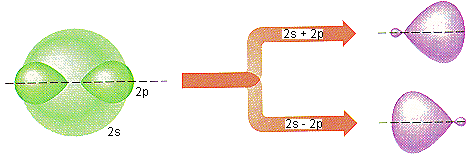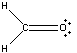Hybrid Atomic Orbitals

Hybrid Atomic Orbitals

Hybrid Atomic Orbitals

It is difficult to explain the shapes of even the simplest molecules with atomic orbitals. A solution to this problem was proposed by Linus Pauling, who argued that the valence orbitals on an atom could be combined to form hybrid atomic orbitals.

The geometry of a BeF2 molecule can be explained, for example, by mixing the 2s orbital on the beryllium atom with one of the 2p orbitals to form a set of sp hybrid orbitals that point in opposite directions, as shown in the figure below. One of the valence electrons on the beryllium atom is then placed in each of these orbitals, and these orbitals are allowed to overlap with half-filled 2p orbitals on a pair of fluorine atoms to form a linear BeF2 molecule.Pauling also showed that the geometry of molecules such as BF3 and the CO32- ion could be explained by mixing a 2s orbital with both a 2px and a 2py orbital on the central atom to form three sp2 hybrid orbitals that point toward the corners of an equilateral triangle. When he mixed a 2s orbital with all three 2p orbitals (2px, 2py and 2pz), Pauling obtained a set of four sp3 orbitals that are oriented toward the corners of a tetrahedron. These sp3 hybrid orbitals are ideal for explaining the geometries of tetrahedral molecules such as CH4 or the SO42- ion.

The hybrid atomic orbital model can be extended to molecules whose shapes are based on trigonal bipyramidal or octahedral distributions of electrons by including valence-shell d orbitals. Pauling showed that when the 3dz2 orbital is mixed with the 3s, 3px, 3py and 3pz orbitals on an atom, the resulting sp3d hybrid orbitals point toward the corners of a trigonal bipyramid. When both the 3dz2 and 3dx2-y2 orbitals are mixed with the 3s, 3px, 3py and 3pz orbitals, the result is a set of six sp3d2 hybrid orbitals that point toward the corners of an octahedron.Geometries of Hybrid Orbitals

The geometries of the five different sets of hybrid atomic orbitals (sp, sp2, sp3, sp3d and sp3d2) are shown in the figure below.The Relationship Between the Distribution of Electrons in an Atom and the Hybridization of That Atom

The relationship between hybridization and the distribution of electrons in the valence shell of an atom is summarized in the table below.

 Number of Places Where Electrons are Found Molecular Geometry Hybridization Examples 2 linear sp BeF2, CO2 3 trigonal planar sp2 BF3, CO32- 4 tetrahedral sp3 CH4, SO42- 5 trigonal bipyramidal sp3d PF5 6 octahedral sp3d2 SF6

 Practice Problem 8:Use the table above to determine the hybridization of the central atom in the following compounds.Click here to check your answer to Practice Problem 8Molecules with Double and Triple Bonds

The hybrid atomic orbital model can also be used to explain the formation of double and triple bonds.

Example: Let's consider the bonding in formaldehyde (H2CO), for example, which has the following Lewis structure.There are three places where electrons can be found in the valence shell of both the carbon and oxygen atoms in this molecule. As a result, the VSEPR theory predicts that the valence electrons on these atoms will be oriented toward the corners of an equilateral triangle. Let's assume, for the sake of argument, that the formaldehyde molecule lies in the XY plane of a coordinate system. We can create a set of sp2 hybrid orbitals on the carbon and oxygen atoms that lie in this plane by mixing the 2s, 2px and 2py orbitals on each atom.

There are four valence electrons on a neutral carbon atom. One of these electrons is placed in each of the three sp2 hybrid orbitals. The fourth electron is placed in the 2pz orbital that wasn't used during hybridization.

There are six valence electrons on a neutral oxygen atom. A pair of these electrons is placed in each of two of the sp2 hybrid orbitals. One electron is then placed in the sp2 hybrid orbital that points toward the carbon atom, and another is placed in the unhybridized 2pz orbital.

The C-H bonds are formed when the electrons in two of the sp2 hybrid orbitals on carbon interact with a 1s electron on a hydrogen atom, as shown in the figure below.. A C-O bond is formed when the electron in the other sp2 hybrid orbital on carbon interacts with the unpaired electron in the sp2 hybrid orbital on the oxygen atom. These bonds are called sigma (s) bonds because they look like an s orbital when viewed along the bond.The electron in the 2pz orbital on the carbon atom then interacts with the electron in the 2pz orbital on the oxygen atom to form a second covalent bond between these atoms. This is called a pi (p) bond because its looks like a p orbital when viewed along the bond.

Double bonds occur most often in compounds that contain C, N, O, P, or S atoms. There are two reasons for this. First, double bonds by their very nature are covalent bonds. They are therefore most likely to be found among the elements that form covalent compounds. Second, the interaction between pz orbitals to form a p bond requires that the atoms come relatively close together, so these bonds tend to be the strongest for atoms that are relatively small.